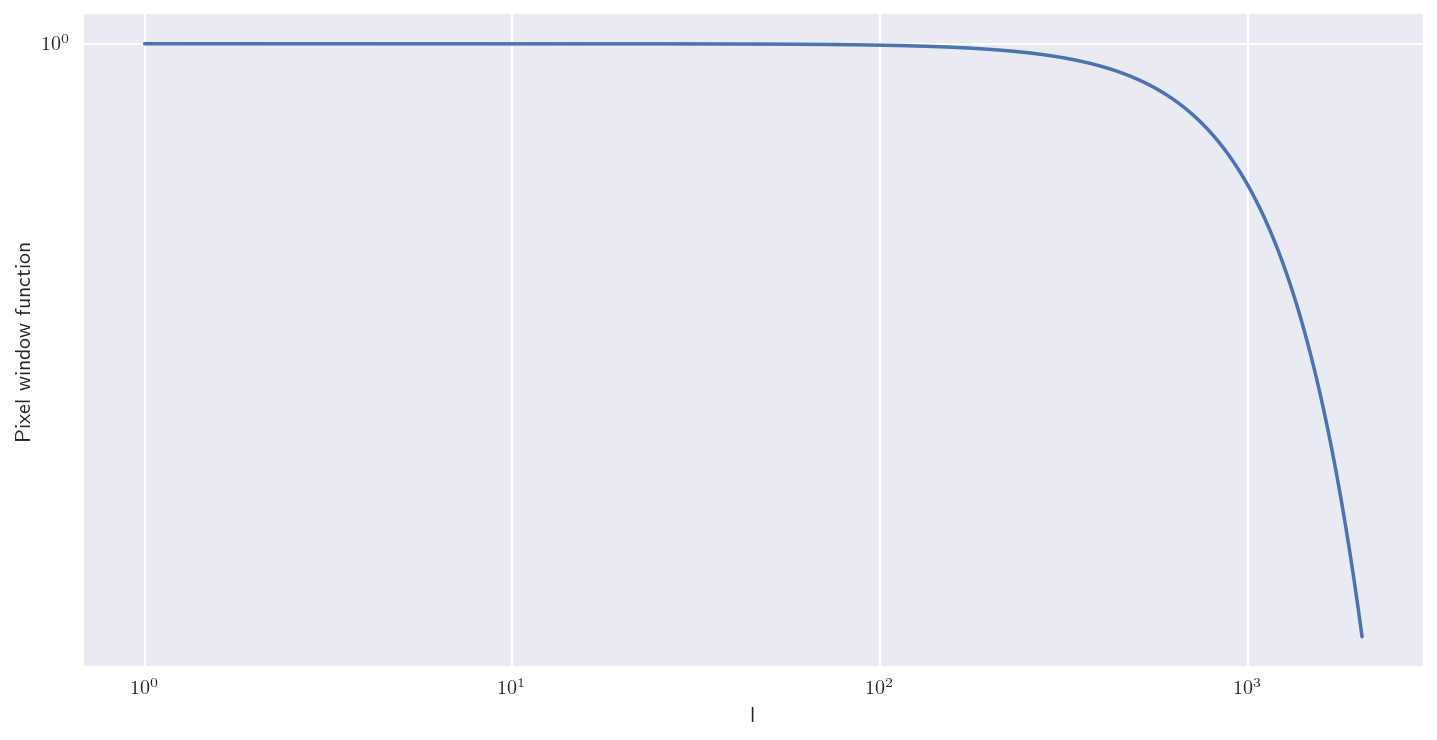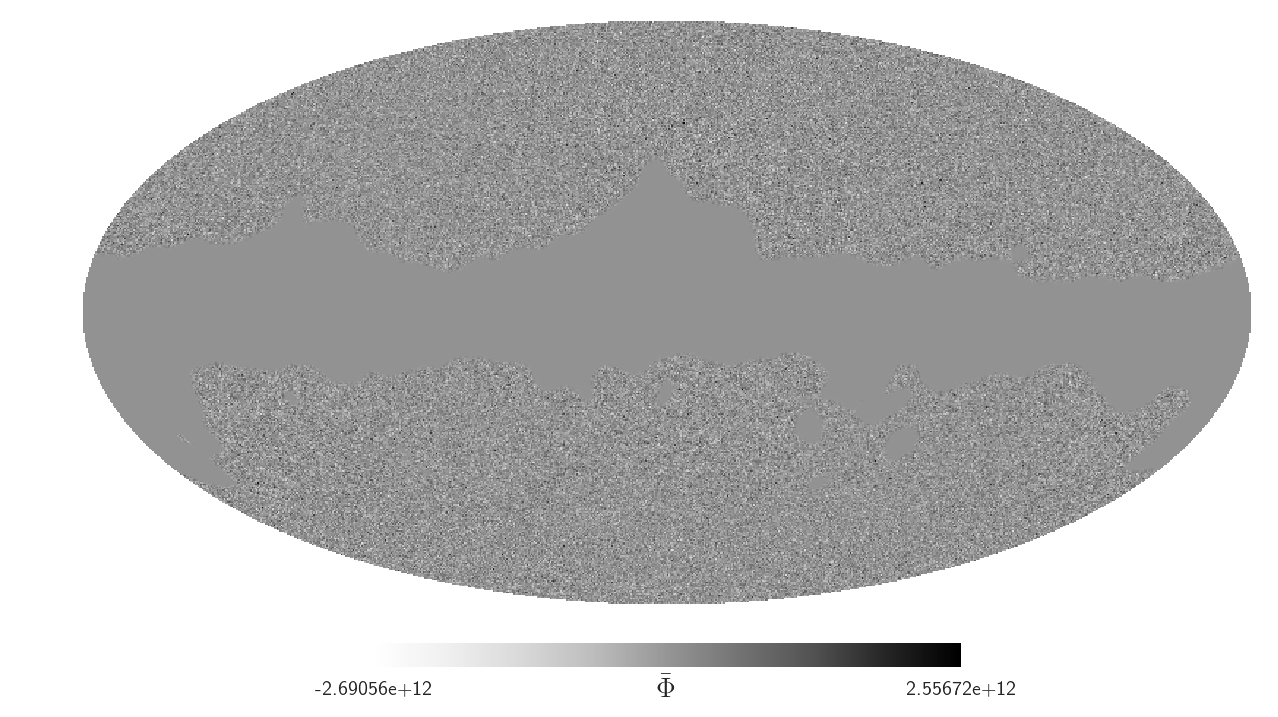I have recently been working on the cross-correlation of CIB intensity and CMB lensing from the Planck data. I've had a few hiccups with the format of the lensing data and thus decided to document this properly in a notebook, just in case others ran into similar issues. I use the PR1 and PR2 data release, each of which has a slightly different format.

The goal is to understand the data format, units, and conventions to use this map for CIB-lensing cross correlations. Furthermore, I demonstrate how to deal with maps, $a_{lm}$, and $C_l$ in healpy while also dealing with sky fractions and window functions.

A big thanks goes to Duncan Hanson, author of the Planck 2015 lensing paper, for help with the data format and for clarifying various issues that I ran into.

In :
%load_ext autoreload
%matplotlib inline
%config InlineBackend.figure_format = 'retina'

In :
import numpy as np
import healpy as hp
import seaborn as sns
import matplotlib.pyplot as plt
from matplotlib import rcParams
rcParams['figure.figsize'] = (12, 6)
rcParams['text.usetex'] = True

from joblib import Memory
mem = Memory(cachedir='.joblib')

import warnings
warnings.filterwarnings('ignore')


We start by defining some parameters for this notebook. Update the datapath if you want to download the data yourself and re-run the notebook.

In :
NSIDE = 2048
LMAX = 2048  # The lensing data are limited to 10 < l < 2048
l = np.arange(LMAX)
datapath = '/users/dlenz/projects/planck/data/cmb_lensing/'
pixfunc = hp.pixwin(NSIDE)


The pixel window function can be generated with healpy and is used to correct the $C_l$ for the finite pixelsize, which we demonstrate later.

In :
plt.loglog(l, pixfunc[:LMAX])
plt.xlabel('l')
plt.ylabel('Pixel window function')

Out:
<matplotlib.text.Text at 0x10aba5240>We furthermore create a helper to smooth the mask, also caching the result.

In :
@mem.cache
smoothing_scale = 2.  # in degree


# PR1 from 2013¶

The PR1 data are taken from here and described in Planck collaboration (2013) XVII. The data product is also accessible from the Planck Legacy Archive (PLA).

In :
pr1_ext1 = fits.getdata(datapath + 'pr1/COM_CompMap_Lensing_2048_R1.10.fits', ext=1)
phibar = hp.reorder(pr1_ext1['PHIBAR'], n2r=True)

In :
# Note that smoothing will take a while (~10 minutes) with this high-resolution mask and the large kernel

WARNING:root:[MemorizedFunc(func=<function smooth_mask at 0x10ac4cae8>, cachedir='.joblib/joblib')]: Clearing cache .joblib/joblib/__main__--Users-dlenz-projects-cibxphi-notebooks-__ipython-input__/smooth_mask

________________________________________________________________________________
Sigma is 50.959308 arcmin (0.014823 rad)
-> fwhm is 120.000000 arcmin
Sigma is 0.000000 arcmin (0.000000 rad)
-> fwhm is 0.000000 arcmin


Inspect the lensing potential:

In :
hp.mollview(phibar * mask_pr1_apod, title='', unit=r'$\bar\Phi$')Second, also load the response function $R_l^{\Phi\Phi}$ and the noise term $N_l^{\Phi\Phi}$. These are later used to correct the derived angular power spectrum of the lensing potential.

In :
pr1_ext2 = fits.getdata(datapath + 'pr1/COM_CompMap_Lensing_2048_R1.10.fits', ext=2)
rlpp = pr1_ext2['RLPP']
nlpp = pr1_ext2['NLPP']

In :
plt.plot(rlpp, label=r'$R_l^{\Phi\Phi}$')
plt.plot(nlpp, label=r'$N_l^{\Phi\Phi}$')
plt.loglog()

# labels & legend
plt.legend()